# Plot Makeover #2

Making a dodged-stacked hybrid bar plot in {ggplot2}

June Choe (University of Pennsylvania Linguistics)https://live-sas-www-ling.pantheon.sas.upenn.edu/
11-08-2020

This is the second installment of plot makeover where I take a plot in the wild and make very opinionated modifications to it.

## Before

Our plot-in-the-wild comes from , a paper on statistical word learning. The plot that I’ll be looking at here is Figure 2, a bar plot of accuracy in a 3-by-3 experimental design.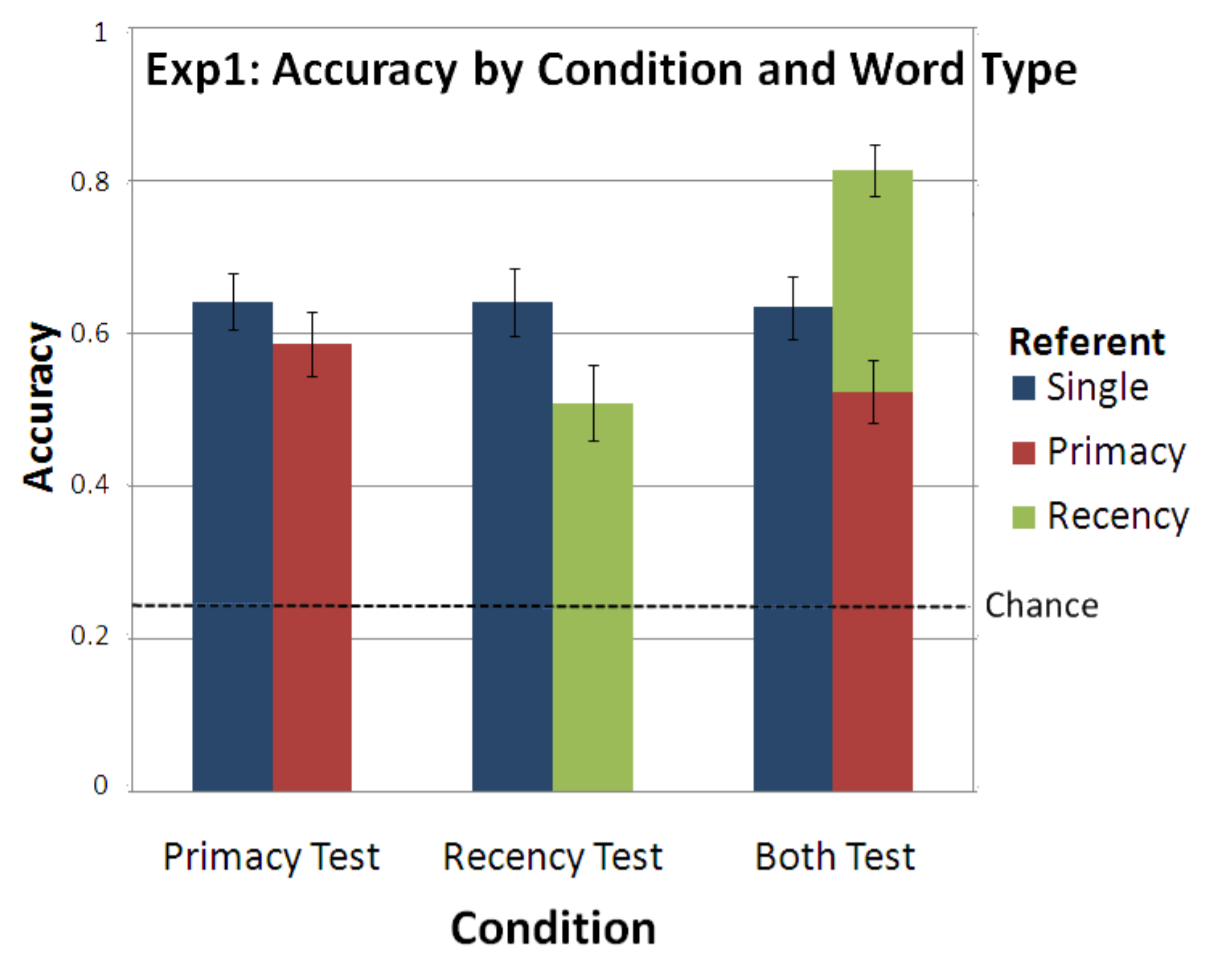Figure 1: Plot from Yurovsky and Yu (2008)

As you might notice, there’s something interesting going on in this bar plot. It looks like the red and green bars stack together but dodge from the blue bar. It’s looks a bit weird for me as someone who mainly uses {ggplot2} because this kind of a hybrid design is not explicitly supported in the API.

For this plot makeover, I’ll leave aside the issue of whether having a half-stacked, half-dodged bar plot is a good idea.1 In fact, I’m not even gonna focus much on the “makeover” part. Instead I’m just going to take a shot at recreating this plot (likely made in MATLAB with post-processing in PowerPoint) in {ggplot2}.

## My Plan

Again, my primary goal here is replication. But I do want to touch up on some aesthetics while I’m at it.

Major Changes:

• Move the title to above the plot

• Move the legend inside the plot

• Move/remove the y-axis title so it’s not vertically aligned

Minor Changes:

• Remove grid lines

• Put y-axis in percentages

• Add white borders around the bars for clearer color contrast

## After

### First draft

For a first pass on the makeover, I wanted to get the hybrid design right.

The plot below isn’t quite there in terms of covering everything I laid out in my plan, but it does replicate the bar plot design specifically.

Plot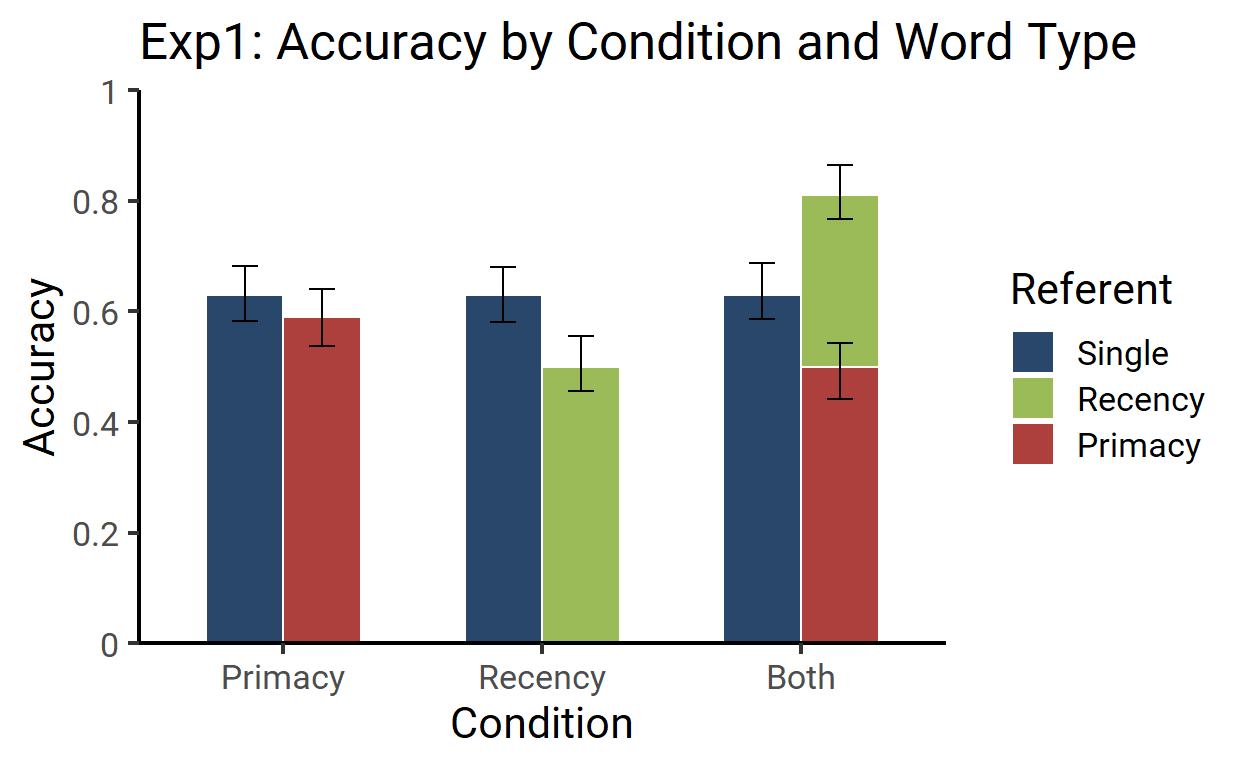Code

``````library(tidyverse)
library(extrafont)

df <- tribble(
~Condition, ~Referent, ~Accuracy,
"Primacy",  "Single",  0.63,
"Primacy",  "Primacy", 0.59,
"Recency",  "Single",  0.63,
"Recency",  "Recency", 0.5,
"Both",     "Single",  0.63,
"Both",     "Primacy", 0.5,
"Both",     "Recency", 0.31
) %>%
mutate(
error_low = runif(7, .04, .06),
error_high = runif(7, .04, .06),
Condition_name = factor(Condition, levels = c("Primacy", "Recency", "Both")),
Condition = as.numeric(Condition_name),
Referent = factor(Referent, levels = c("Single", "Recency", "Primacy")),
left = Referent == "Single",
color = case_when(
Referent == "Single" ~ "#29476B",
Referent == "Recency" ~ "#9BBB58"
)
)

ggplot(mapping = aes(x = Condition, y = Accuracy, fill = color)) +
geom_col(
data = filter(df, left),
width = .3,
color = "white",
position = position_nudge(x = -.3)
) +
geom_errorbar(
aes(ymin = Accuracy - error_low, ymax = Accuracy + error_high),
data = filter(df, left),
width = .1,
position = position_nudge(x = -.3)
) +
geom_col(
data = filter(df, !left),
color = "white",
width = .3,
) +
geom_errorbar(
aes(y = y, ymin = y - error_low, ymax = y + error_high),
data = filter(df, !left) %>%
group_by(Condition) %>%
mutate(y = accumulate(Accuracy, sum)),
width = .1
) +
scale_fill_identity(
labels = levels(df\$Referent),
guide = guide_legend(title = "Referent")
) +
scale_x_continuous(
breaks = 1:3 - .15,
labels = levels(df\$Condition_name),
expand = expansion(.1)
) +
scale_y_continuous(
breaks = scales::pretty_breaks(6),
labels = str_remove(scales::pretty_breaks(6)(0:1), "\\.0+"),
limits = 0:1,
expand = expansion(0)
) +
labs(
title = "Exp1: Accuracy by Condition and Word Type"
) +
theme_classic(
base_family = "Roboto",
base_size = 16
)
``````

As you might guess from my two calls to `geom_col()` and `geom_errorbar()`, I actually split the plotting of the bars into two parts. First I drew the blue bars and their errorbars, then I drew the green and red bars and their errorbars.

Effectively, the above plot is a combination of these two:2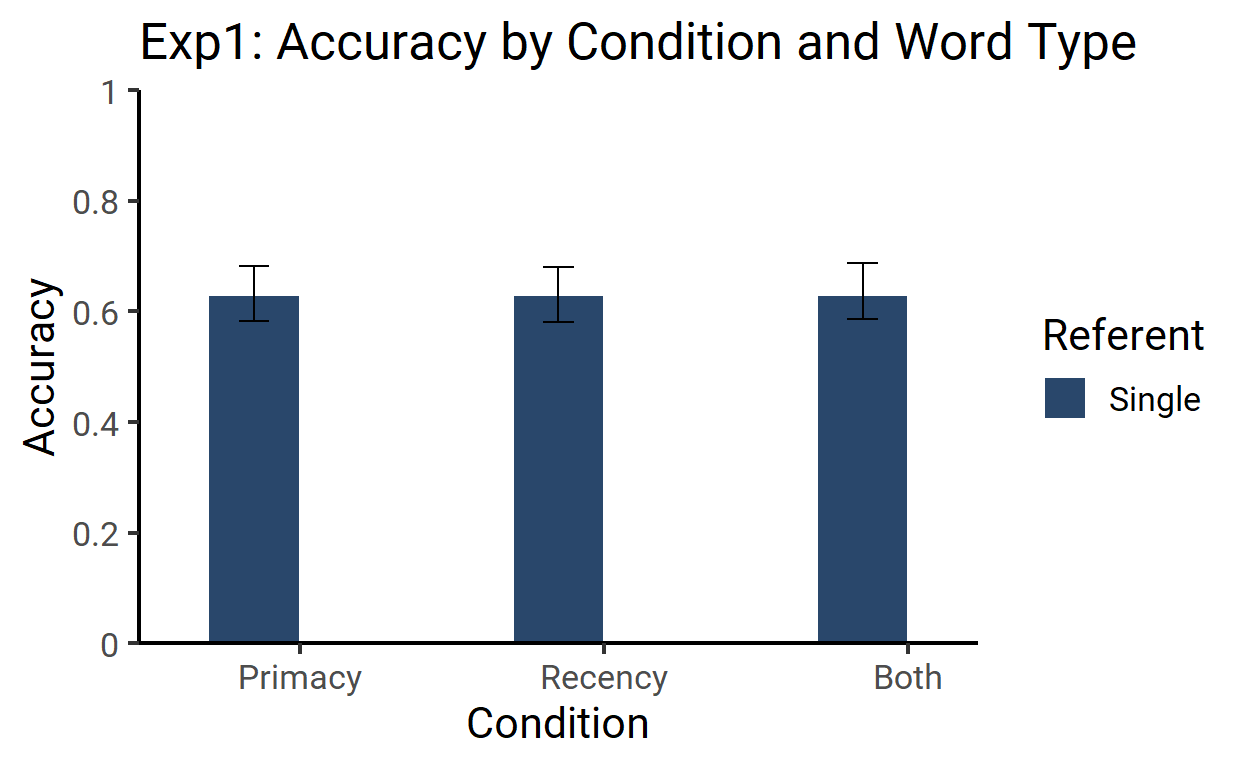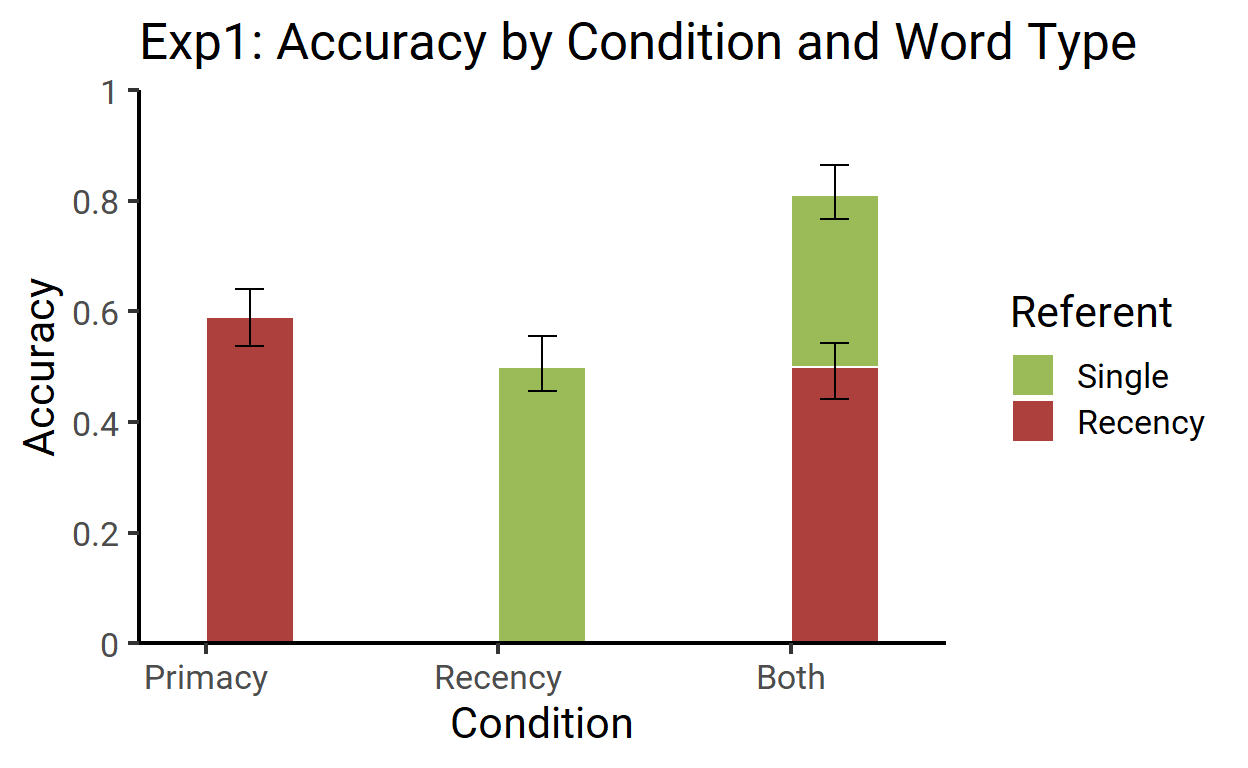A bit hacky, I guess, but it works!

### Final touch-up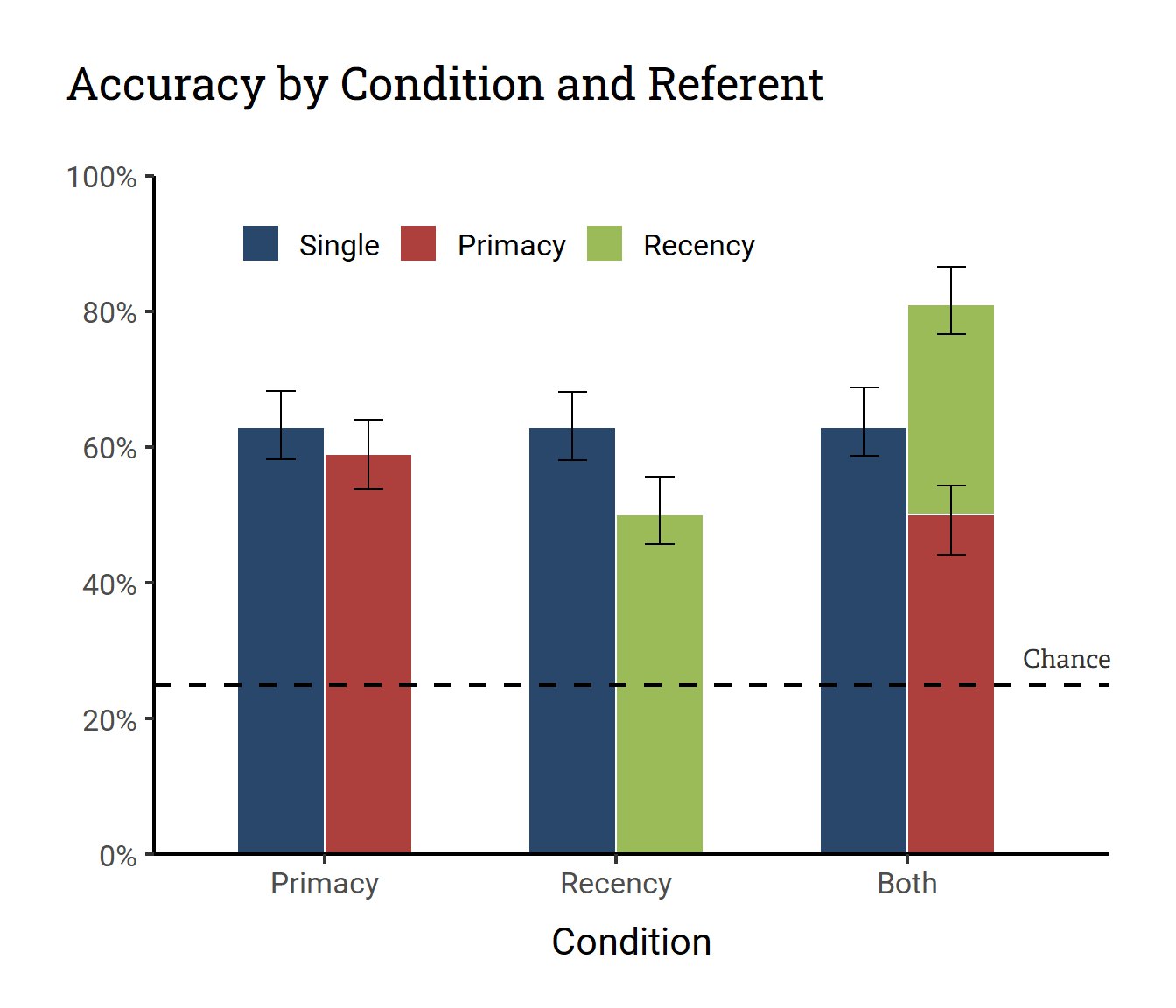``````ggplot(mapping = aes(x = Condition, y = Accuracy, fill = color)) +
geom_col(
data = filter(df, left),
width = .3,
color = "white",
position = position_nudge(x = -.3),
) +
geom_errorbar(
aes(ymin = Accuracy - error_low, ymax = Accuracy + error_high),
data = filter(df, left),
width = .1,
position = position_nudge(x = -.3)
) +
geom_col(
data = filter(df, !left),
color = "white",
width = .3,
) +
geom_errorbar(
aes(y = y, ymin = y - error_low, ymax = y + error_high),
data = filter(df, !left) %>%
group_by(Condition) %>%
mutate(y = accumulate(Accuracy, sum)),
width = .1
) +
geom_hline(
aes(yintercept = .25),
linetype = 2,
size = 1,
) +
geom_text(
aes(x = 3.4, y = .29),
label = "Chance",
color = "grey20",
inherit.aes = FALSE
) +
scale_fill_identity(
labels = c("Single", "Primacy", "Recency"),
guide = guide_legend(
title = NULL,
direction = "horizontal",
override.aes = list(fill = c("#29476B", "#AD403D", "#9BBB58"))
)
) +
scale_x_continuous(
breaks = 1:3 - .15,
labels = levels(df\$Condition_name),
expand = expansion(c(.1, .05))
) +
scale_y_continuous(
breaks = scales::pretty_breaks(6),
labels = scales::percent_format(1),
limits = 0:1,
expand = expansion(0)
) +
labs(
title = "Accuracy by Condition and Referent",
y = NULL
) +
theme_classic(
base_family = "Roboto",
base_size = 16
) +
theme(
plot.title.position = "plot",
plot.title = element_text(
family = "Roboto Slab",
margin = margin(0, 0, 1, 0, "cm")
),
legend.position = c(.35, .9),
axis.title.x = element_text(margin = margin(t = .4, unit = "cm")),
plot.margin = margin(1, 1, .7, 1, "cm")
)
``````
Yurovsky, Daniel, and C. Yu. 2008. Mutual Exclusivity in Cross-Situational Statistical Learning. https://dll.sitehost.iu.edu/papers/Yurovsky_cs08.pdf.